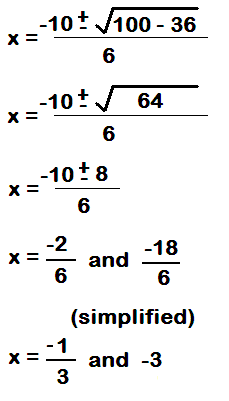Use the quadratic formula to find the roots of the general quadratic equation ax2+bx+c=0 with this Quadratic Equation Calculator.

a: b: c:

Fill in the constants (a, b, and c) from the quadratic equation
ax2+bx+c=0, to solve for x.

### What is the quadratic equation?

The quadratic equation is a mathematical equation that is written
in the form ax2+bx+c=0.

The quadratic formula is a formula that is used to find the roots

To calculate the roots (x) of a quadratic equation, use the quadratic
formula:Example: For a quadratic equation 3x2+10x+3=0, solve using the